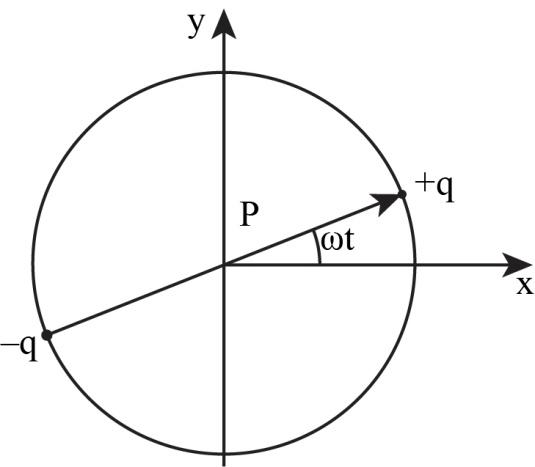×
Get Full Access to Introduction To Electrodynamics - 4 Edition - Chapter 11 - Problem 4p
Get Full Access to Introduction To Electrodynamics - 4 Edition - Chapter 11 - Problem 4p

×

# A rotating electric dipole can be thought of as theISBN: 9780321856562 45

## Solution for problem 4P Chapter 11

Introduction to Electrodynamics | 4th Edition

• Textbook Solutions
• 2901 Step-by-step solutions solved by professors and subject experts
• Get 24/7 help from StudySoup virtual teaching assistantsIntroduction to Electrodynamics | 4th Edition

4 5 1 259 Reviews
11
3
Problem 4P

Problem 4P

A rotating electric dipole can be thought of as the superposition of two oscillating dipoles, one along the x axis and the other along the y axis (Fig. 11.7), with the latter out of phase by 90˚:Using the principle of superposition and Eqs. 11.18 and 11.19 (perhaps in the form suggested by Prob. 11.2), find the fields of a rotating dipole. Also find the Poynting vector and the intensity of the radiation. Sketch the intensity profile as a function of the polar angle θ, and calculate the total power radiated. Does the answer seem reasonable? (Note that power, being quadratic in the fields, does not satisfy the superposition principle. In this instance, however, it seems to. How do you account for this?)

Reference equation 11.18Reference equation 11.19Reference prob 11.2

Equation 11.14 can be expressed in “coordinate-free” form by writingDo so, and likewise for Eqs. 11.17, 11.18. 11.19, and 11.21.

Step-by-Step Solution:

Problem 4P

A rotating electric dipole can be thought of as the superposition of two oscillating dipoles, one along the x axis and the other along the y axis (Fig. 11.7), with the latter out of phase by:Using the principle of superposition and Eqs. 11.18 and 11.19 (perhaps in the form suggested by Prob. 11.2), find the fields of a rotating dipole. Also find the Poynting vector and the intensity of the radiation. Sketch the intensity profile as a function of the polar angle ?, and calculate the total power radiated. Does the answer seem reasonable? (Note that power, being quadratic in the fields, does not satisfy the superposition principle. In this instance, however, it seems to. How do you account for this?)

Reference equation 11.18Reference equation 11.19Reference prob 11.2

Equation 11.14 can be expressed in “coordinate-free” form by writing. Do so, and likewise for Eqs. 11.17, 11.18. 11.19, and 11.21.

Step by Step Solution

Step 1 of 8

The expression for the gradient of variable f in the polar coordinates can be written as:The expression for the potential in terms of Cartesian coordinates can be written as:The expression for the dipole moment can be written as:Step 2 of 8

Step 3 of 8

##### ISBN: 9780321856562

Introduction to Electrodynamics was written by and is associated to the ISBN: 9780321856562. The full step-by-step solution to problem: 4P from chapter: 11 was answered by , our top Physics solution expert on 07/18/17, 05:41AM. The answer to “A rotating electric dipole can be thought of as the superposition of two oscillating dipoles, one along the x axis and the other along the y axis (Fig. 11.7), with the latter out of phase by 90?: Using the principle of superposition and Eqs. 11.18 and 11.19 (perhaps in the form suggested by Prob. 11.2), find the fields of a rotating dipole. Also find the Poynting vector and the intensity of the radiation. Sketch the intensity profile as a function of the polar angle ?, and calculate the total power radiated. Does the answer seem reasonable? (Note that power, being quadratic in the fields, does not satisfy the superposition principle. In this instance, however, it seems to. How do you account for this?)Reference equation 11.18 Reference equation 11.19 Reference prob 11.2Equation 11.14 can be expressed in “coordinate-free” form by writing Do so, and likewise for Eqs. 11.17, 11.18. 11.19, and 11.21.” is broken down into a number of easy to follow steps, and 151 words. Since the solution to 4P from 11 chapter was answered, more than 456 students have viewed the full step-by-step answer. This textbook survival guide was created for the textbook: Introduction to Electrodynamics , edition: 4. This full solution covers the following key subjects: Superposition, equation, reference, power, prob. This expansive textbook survival guide covers 12 chapters, and 550 solutions.

Unlock Textbook Solution